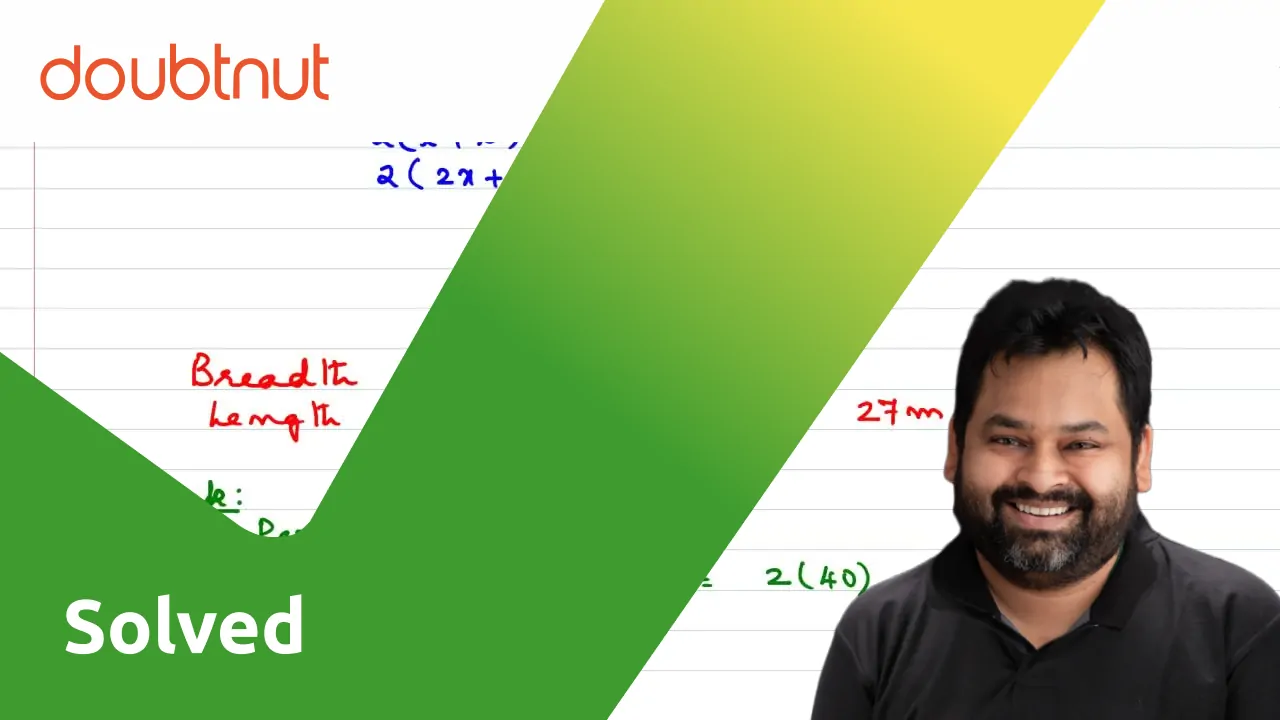Doubtnut App - Get 50 Lakh+ video solutions better than GoogleInstall Now

# The perimeter of a rectangle is 80 metres and its length is one metre more than twice the breadth. What are its length and breadth?Solution in Malayalam

Text Solution
Verified by Experts

## Ans: Let.s take.breadth of the rectangle be x. length =2x+1 Given that perimeter.be 80 metres 2(2x+1+x)=80 3x+1=80+2 That is, 3x+1=40 3x=40−1=39 x=393⋅=13~m That is, breadth of the rectangle =13~m length =2×13+1=27~m